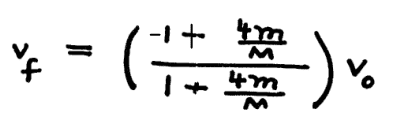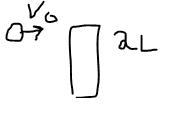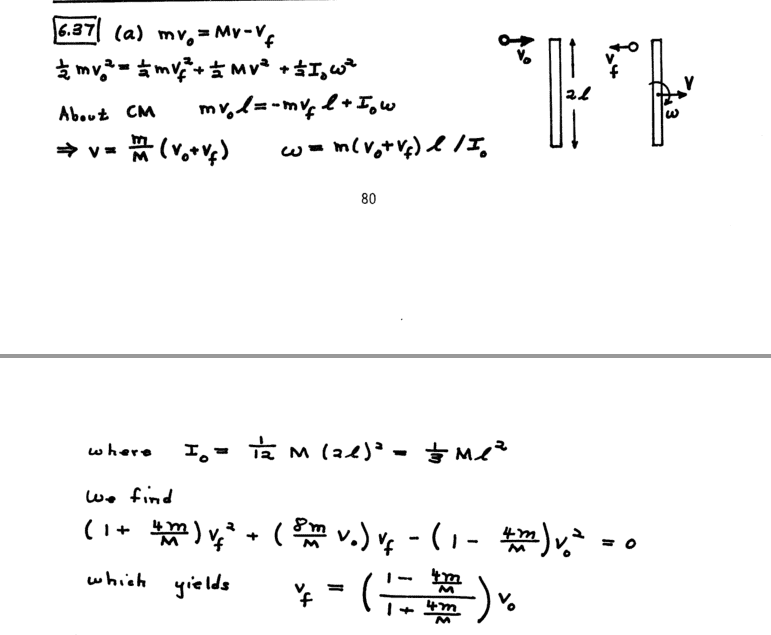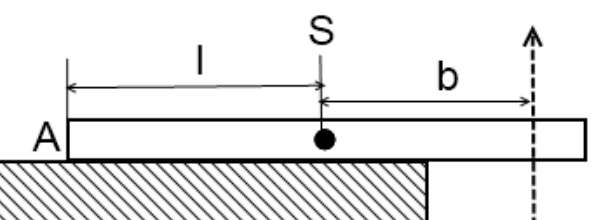# Collision, angular momentum and energy

• LCSphysicist
Yes, but in this case it is rather surprising that they reversed the sign convention for vf. Which way the ball goes after collision depends on how its mass compares with M/4, so it would have been far more natural to have kept the positive direction the same.f

#### LCSphysicist

Homework Statement
A plank of length 2l and mass M lies on a frictionless plane.
A ball of mass m a nd speed Vo strikes its end as shown. Find the final
velocity of the ball, vf, assuming that mechanical energy is conserved
and that vf is along the original line of motion
Relevant Equations
All below.
IS my solution right? Comparing with the other solutions, the answer just exchange the signals, i don't know why,THats what ifound.

And here is the three equations:
{i use the point which occurs the collision}
Lo = Lf >>

0 = Iw + M*Vcm(block)

Eg = ct>

mvo² = mvf² + MVcm² + Iw²

I = ml²/3

P = cte

mvo = mvf + MVcm

I just assumed that xyz dextrogira, i thought that putting by this way, the math would implies the correct values, that is, we would find w<0, and the others values. Apparently i am wrong in something, where?

a picture would be good

a picture would be goodApparently i am wrong in something, where?
I get the same answer you do.

I get the same answer you do.As you can see, in our answer the numerator has signals exchanged.

As you can see, in our answer the numerator has signals exchanged.
You have a different sign convention for ##v_0## and ##v_f##. What's up with that? If you are trying to explain a sign error in the answer, the very first place to look is at the sign conventions.

You have a different sign convention for ##v_0## and ##v_f##. What's up with that? If you are trying to explain a sign error in the answer, the very first place to look is at the sign conventions.
Yes, but in this case it is rather surprising that they reversed the sign convention for vf. Which way the ball goes after collision depends on how its mass compares with M/4, so it would have been far more natural to have kept the positive direction the same.

•jbriggs444
Sorry for offtop, perhaps somebody will be interested.
I remember a much funny problem of the same type. A plank of mass ##m## and of length ##2l## lies on a smooth horizontal table as it is shown at the picture. Here ##S## is the center of mass. A bullet punches through the plank (dotted arrow) and loses linear momentum ##p##. Find the angular velocity of the plank right after the punch. It is not so trivial as it looks from the first glance.•etotheipi
Sorry for offtop, perhaps somebody will be interested.
I remember a much funny problem of the same type. A plank of mass ##m## and of length ##2l## lies on a smooth horizontal table as it is shown at the picture. Here ##S## is the center of mass. A bullet punches through the plank (dotted arrow) and loses linear momentum ##p##. Find the angular velocity of the plank right after the punch. It is not so trivial as it looks from the first glance.

View attachment 264805
Depends whether b>l/3, right?

Depends whether b>l/3, right?
yes :)

Yes, but in this case it is rather surprising that they reversed the sign convention for vf. Which way the ball goes after collision depends on how its mass compares with M/4, so it would have been far more natural to have kept the positive direction the same.
1. Why do they reverse the sign convention for vf when it is a velocity with a direction.
2. Doesn't it say that " assuming that mechanical energy is conserved
and that vf is along the original line of motion "?
3. Are vector arrows meant to be drawn on vector quantities?
4. If we just draw vector arrows on the equation that OP supplies, is there any need for changing the sign now?

Sorry for offtop, perhaps somebody will be interested.
I remember a much funny problem of the same type. A plank of mass ##m## and of length ##2l## lies on a smooth horizontal table as it is shown at the picture. Here ##S## is the center of mass. A bullet punches through the plank (dotted arrow) and loses linear momentum ##p##. Find the angular velocity of the plank right after the punch. It is not so trivial as it looks from the first glance.

View attachment 264805

Help, I want a solution.

Depends whether b>l/3, right?

?

Why do they reverse the sign convention for vf when it is a velocity with a direction.
I assume whoever wrote that solution considered rebound as the expected behaviour, but there is no good reason.
Doesn't it say that " assuming that mechanical energy is conserved
and that vf is along the original line of motion "?
Yes, but being along the same line is not quite the same as in the same direction.
Are vector arrows meant to be drawn on vector quantities?
Yes, but since the velocities are all in the same line there's no problem working with the scalar coefficient of the velocity in the chosen unit vector direction.
If we just draw vector arrows on the equation that OP supplies, is there any need for changing the sign now?
Not sure what you are asking.
If you mean ##\vec {v_f}=\frac{1-\frac{4m}M}{1+\frac{4m}M}\vec{v_0}##, that would simply be wrong.

•jbriggs444
1. Why do they reverse the sign convention for vf when it is a velocity with a direction.
You are asking us to guess about a decision made by someone else. I do not agree with that decision, but I can try to defend it anyway.

Often, one will try to choose a sign convention so that all of the numbers in the problem will be positive. So if one expects the initial velocity to be toward the right and the final velocity toward the left then one might want to choose a sign convention so that positive values of ##v_0## are rightward while positive values of ##v_f## are leftward.

Of course, this means that when those values are combined in an equation, the difference in sign must be accounted for. So instead of writing down a conservation of linear momentum equation like$$mv_0 + Mv_0 = mv_f + MV_f$$we have to write$$mv_0 + MV_0 = -mv_f + MV_f$$.

If we do not know, when initially approaching the problem, what direction the final velocity is going to have, it is more sensible to use the same sign convention (or, more generally, the same coordinate axes) for everything. If that means that the numeric velocity comes out negative, so be it.
2. Doesn't it say that " assuming that mechanical energy is conserved
and that vf is along the original line of motion "?
Yes. But lines extend in two directions. That statement leaves the sign convention one will use to report the final velocity undefined.
3. Are vector arrows meant to be drawn on vector quantities?
Arrows. Or boldface. When working in one dimension, it can be somewhat pointless.
4. If we just draw vector arrows on the equation that OP supplies, is there any need for changing the sign now?
One still has to pay attention to sign conventions. Sticking a graphic enhancement on the equations does not change that.

When dealing with vectors, the sign convention amounts to a choice of a set of basis vectors for the vector space. Those fancy words mean picking a direction for your coordinate axes. If you have vector ##\vec{v_0}## expressed using a coordinate system where positive x points rightward and vector ##\vec{v_f}## using a coordinate system where positive x points leftward then you will have to include a minus sign in your equations when referring to x-coordinates using the other coordinate system. That minus sign amounts to a coordinate system transformation so that all of the coordinate values in an equation are using the same system.

Last edited:
Help, I want a solution.

?
Don't worry about wrobel's post. It is an entirely different, but quite interesting, question.

I assume whoever wrote that solution considered rebound as the expected behaviour, but there is no good reason.

Yes, but being along the same line is not quite the same as in the same direction.

Yes, but since the velocities are all in the same line there's no problem working with the scalar coefficient of the velocity in the chosen unit vector direction.

Not sure what you are asking.
If you mean ##\vec {v_f}=\frac{1-\frac{4m}M}{1+\frac{4m}M}\vec{v_0}##, that would simply be wrong.
You are asking us to guess about a decision made by someone else. I do not agree with that decision, but I can try to defend it anyway.

Often, one will try to choose a sign convention so that all of the numbers in the problem will be positive. So if one expects the initial velocity to be toward the right and the final velocity toward the left then one might want to choose a sign convention so that positive values of ##v_0## are rightward while positive values of ##v_f## are leftward.

Of course, this means that when those values are combined in an equation, the difference in sign must be accounted for. So instead of writing down a conservation of linear momentum equation like$$mv_0 + Mv_0 = mv_f + MV_f$$we have to write$$mv_0 + MV_0 = -mv_f + MV_f$$.

If we do not know, when initially approaching the problem, what direction the final velocity is going to have, it is more sensible to use the same sign convention (or, more generally, the same coordinate axes) for everything. If that means that the numeric velocity comes out negative, so be it.

Yes. But lines extend in two directions. That statement leaves the sign convention one will use to report the final velocity undefined.

Arrows. Or boldface. When working in one dimension, it can be somewhat pointless.

One still has to pay attention to sign conventions. Sticking a graphic enhancement on the equations does not change that.

When dealing with vectors, the sign convention amounts to a choice of a set of basis vectors for the vector space. Those fancy words mean picking a direction for your coordinate axes. If you have vector ##\vec{v_0}## expressed using a coordinate system where positive x points rightward and vector ##\vec{v_f}## using a coordinate system where positive x points leftward then you will have to include a minus sign in your equations when referring to x-coordinates using the other coordinate system. That minus sign amounts to a coordinate system transformation so that all of the coordinate values in an equation are using the same system.

For Question 4, what I mean is doesn't V with an arrow on top indicate both magnitude and direction along the defined axis. So from the start when we do V0 and Vf, we simply ignore all signs and write all of them as positive, rather than using the scalar coefficient?

Don't worry about wrobel's post. It is an entirely different, but quite interesting, question.

Don't care if it's different, please show solution.

Don't care if it's different, please show solution.
The trick in the question is you have to consider two possibilities. If the impact is sufficiently close to the rod's mass centre, the rod will rotate relatively slowly, so the end that was on the table will rise off the table. If the impact is sufficiently far from the rod's mass centre, the rod's rotation could be so fast that the end at A would move down into the table; to prevent that there must be a normal impulse at A.

•wrobel
For Question 4, what I mean is doesn't V with an arrow on top indicate both magnitude and direction along the defined axis. So from the start when we do V0 and Vf, we simply ignore all signs and write all of them as positive, rather than using the scalar coefficient?
Yes, hence my answer in post #13.

Yes, hence my answer in post #13.
You say that would be simply wrong? Why would it be wrong?

You say that would be simply wrong? Why would it be wrong?
When working in vectors, unless otherwise stated, they should all be in the same coordinate system.
Consider e.g. m tends to zero. That vector equation has ##\vec {v_f}## tending to ##\vec{v_0}## instead of to ##-\vec{v_0}##. When m is very small it should just rebound, hardly disturbing M.

When working in vectors, unless otherwise stated, they should all be in the same coordinate system.
Consider e.g. m tends to zero. That vector equation has ##\vec {v_f}## tending to ##\vec{v_0}## instead of to ##-\vec{v_0}##. When m is very small it should just rebound, hardly disturbing M.

?
Vectors are along the same line. Arrow drawn on top indicates that the direction is included.

I am saying that at the very start of working out, we do not take a -vf and a v0 but just take a vf and v0 with an arrow on top.
Then we end with the original answer OP got which was vf (arrow) = (-1+4m/M)/(1+4m/M) v0 (arrow).
We never bother with what happens, but this would be correct since v0 would be in the different direction from vf, and we end with vf = (1-4m/M)/(1+4m/M) v0 if we bother to make it into scalar form.

?
Vectors are along the same line. Arrow drawn on top indicates that the direction is included.

I am saying that at the very start of working out, we do not take a -vf and a v0 but just take a vf and v0 with an arrow on top.
Then we end with the original answer OP got which was vf (arrow) = (-1+4m/M)/(1+4m/M) v0 (arrow).
We never bother with what happens, but this would be correct since v0 would be in the different direction from vf, and we end with vf = (1-4m/M)/(1+4m/M) v0 if we bother to make it into scalar form.
We seem to be at cross purposes.
In post #13 I wrote that ##\vec {v_f}=\frac{1-\frac{4m}M}{1+\frac{4m}M}\vec{v_0}## would be wrong.
##\vec {v_f}=\frac{-1+\frac{4m}M}{1+\frac{4m}M}\vec{v_0}## would be correct.

•aspodkfpo